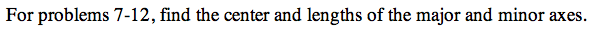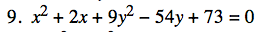### Home > PC > Chapter 13 > Lesson 13.1.2 > Problem13E-9

13E-9.Refer to Example 3 to assist you with this problem.

Complete the Square to put the equation in Standard Form.

$\left(\textit{x}^2+2\textit{x}+1\right)+9\left(\textit{y}^2-6\textit{y}+9\right)=-73+1+9\left(9\right)$